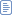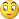#2006年12月16日

## windows消息大全

type

TMsg = packed record

hwnd: HWND; / /窗口句柄

message: UINT; / /消息常量标识符

wParam: WPARAM ; // 32位消息的特定附加信息

lParam: LPARAM ; // 32位消息的特定附加信息

time: DWORD; / /消息创建时的时间

pt: TPoint; / /消息创建时的鼠标位置

end;

hwnd 32位的窗口句柄。窗口可以是任何类型的屏幕对象，因为Win32能够维护大多数可视对象的句柄(窗口、对话框、按钮、编辑框等)。

message 用于区别其他消息的常量值，这些常量可以是Windows单元中预定义的常量，也可以是自定义的常量。

wParam 通常是一个与消息有关的常量值，也可能是窗口或控件的句柄。

lParam 通常是一个指向内存中数据的指针。由于W P a r a m、l P a r a m和P o i n t e r都是3 2位的，

WM_NULL = \$0000;

WM_CREATE = \$0001;

WM_DESTROY = \$0002;

WM_MOVE = \$0003;

WM_SIZE = \$0005;

WM_ACTIVATE = \$0006;

WM_SETFOCUS = \$0007;

WM_KILLFOCUS = \$0008;

WM_ENABLE = \$000A;

WM_SETREDRAW = \$000B;

WM_SETTEXT = \$000C;

WM_GETTEXT = \$000D;

WM_GETTEXTLENGTH = \$000E;

WM_PAINT = \$000F;

WM_CLOSE = \$0010;

WM_QUERYENDSESSION = \$0011;

WM_QUIT = \$0012;

WM_QUERYOPEN = \$0013;

WM_ERASEBKGND = \$0014;

WM_SYSCOLORCHANGE = \$0015;

WM_ENDSESSION = \$0016;

WM_SYSTEMERROR = \$0017;

WM_SHOWWINDOW = \$0018;

WM_ACTIVATEAPP = \$001C;

WM_FONTCHANGE = \$001D;

WM_TIMECHANGE = \$001E;

WM_CANCELMODE = \$001F;

WM_SETCURSOR = \$0020;

WM_MOUSEACTIVATE = \$0021;

WM_CHILDACTIVATE = \$0022;

WM_QUEUESYNC = \$0023;

WM_GETMINMAXINFO = \$0024;

WM_PAINTICON = \$0026;

WM_ICONERASEBKGND = \$0027;

WM_NEXTDLGCTL = \$0028;

WM_SPOOLERSTATUS = \$002A;

WM_DRAWITEM = \$002B;

WM_MEASUREITEM = \$002C;

WM_DELETEITEM = \$002D;

WM_VKEYTOITEM = \$002E;

WM_CHARTOITEM = \$002F;

WM_SETFONT = \$0030;

WM_GETFONT = \$0031;

WM_SETHOTKEY = \$0032;

WM_GETHOTKEY = \$0033;

WM_QUERYDRAGICON = \$0037;

WM_COMPAREITEM = \$0039;

WM_GETOBJECT = \$003D;

WM_COMPACTING = \$0041;

WM_WINDOWPOSCHANGING = \$0046;

WM_WINDOWPOSCHANGED = \$0047;

WM_POWER = \$0048;（适用于16位的windows）

WM_COPYDATA = \$004A;

WM_CANCELJOURNAL = \$004B;

WM_NOTIFY = \$004E;

WM_INPUTLANGCHANGEREQUEST = \$0050;

WM_INPUTLANGCHANGE = \$0051;

WM_TCARD = \$0052;

WM_HELP = \$0053;

WM_USERCHANGED = \$0054;

WM_NOTIFYFORMAT = \$0055;

WM_STYLECHANGING = \$007C;

WM_STYLECHANGED = \$007D;

WM_DISPLAYCHANGE = \$007E;

WM_GETICON = \$007F;

WM_SETICON = \$0080;

WM_NCCREATE = \$0081;

WM_NCDESTROY = \$0082;

WM_NCCALCSIZE = \$0083;

WM_NCHITTEST = \$0084;//移动鼠标，按住或释放鼠标时发生

WM_NCPAINT = \$0085;

WM_NCACTIVATE = \$0086;

WM_GETDLGCODE = \$0087;

WM_NCMOUSEMOVE = \$00A0;

WM_NCLBUTTONDOWN = \$00A1;

WM_NCLBUTTONUP = \$00A2;

WM_NCLBUTTONDBLCLK = \$00A3;

WM_NCRBUTTONDOWN = \$00A4;

WM_NCRBUTTONUP = \$00A5;

WM_NCRBUTTONDBLCLK = \$00A6;

WM_NCMBUTTONDOWN = \$00A7;

WM_NCMBUTTONUP = \$00A8;

WM_NCMBUTTONDBLCLK = \$00A9;

WM_KEYFIRST = \$0100;

WM_KEYDOWN = \$0100;

//按下一个键

WM_KEYUP = \$0101;

//释放一个键

WM_CHAR = \$0102;

//按下某键，并已发出WM_KEYDOWN， WM_KEYUP消息

WM_SYSKEYDOWN = \$0104;

WM_SYSKEYUP = \$0105;

WM_SYSCHAR = \$0106;

WM_KEYLAST = \$0108;

WM_INITDIALOG = \$0110;

WM_COMMAND = \$0111;

WM_SYSCOMMAND = \$0112;

WM_TIMER = \$0113; //发生了定时器事件

WM_HSCROLL = \$0114;

WM_VSCROLL = \$0115;

WM_ENTERIDLE = \$0121;

WM_CHANGEUISTATE = \$0127;

WM_UPDATEUISTATE = \$0128;

WM_QUERYUISTATE = \$0129;

WM_CTLCOLORMSGBOX = \$0132;

WM_CTLCOLOREDIT = \$0133;

WM_CTLCOLORLISTBOX = \$0134;

WM_CTLCOLORBTN = \$0135;

WM_CTLCOLORDLG = \$0136;

WM_CTLCOLORSCROLLBAR= \$0137;

WM_CTLCOLORSTATIC = \$0138;

WM_MOUSEFIRST = \$0200;

WM_MOUSEMOVE = \$0200;

// 移动鼠标

WM_LBUTTONDOWN = \$0201;

//按下鼠标左键

WM_LBUTTONUP = \$0202;

//释放鼠标左键

WM_LBUTTONDBLCLK = \$0203;

//双击鼠标左键

WM_RBUTTONDOWN = \$0204;

//按下鼠标右键

WM_RBUTTONUP = \$0205;

//释放鼠标右键

WM_RBUTTONDBLCLK = \$0206;

//双击鼠标右键

WM_MBUTTONDOWN = \$0207;

//按下鼠标中键

WM_MBUTTONUP = \$0208;

//释放鼠标中键

WM_MBUTTONDBLCLK = \$0209;

//双击鼠标中键

WM_MOUSEWHEEL = \$020A;

WM_MOUSELAST = \$020A;

WM_PARENTNOTIFY = \$0210;

WM_SIZING = 532;

WM_CAPTURECHANGED = 533;

WM_MOVING = 534;

WM_DEVICECHANGE = 537;

WM_IME_STARTCOMPOSITION = \$010D;

WM_IME_ENDCOMPOSITION = \$010E;

WM_IME_COMPOSITION = \$010F;

WM_IME_KEYLAST = \$010F;

WM_IME_SETCONTEXT = \$0281;

WM_IME_NOTIFY = \$0282;

WM_IME_CONTROL = \$0283;

WM_IME_COMPOSITIONFULL = \$0284;

WM_IME_SELECT = \$0285;

WM_IME_CHAR = \$0286;

WM_IME_REQUEST = \$0288;

WM_IME_KEYDOWN = \$0290;

WM_IME_KEYUP = \$0291;

WM_MDICREATE = \$0220;

WM_MDIDESTROY = \$0221;

WM_MDIACTIVATE = \$0222;

WM_MDIRESTORE = \$0223;

WM_MDINEXT = \$0224;

WM_MDIMAXIMIZE = \$0225;

WM_MDITILE = \$0226;

WM_MDIICONARRANGE = \$0228;

WM_MDIGETACTIVE = \$0229;

WM_ENTERSIZEMOVE = \$0231;

WM_EXITSIZEMOVE = \$0232;

WM_DROPFILES = \$0233;

WM_MOUSEHOVER = \$02A1;

WM_MOUSELEAVE = \$02A3;

WM_CUT = \$0300;

WM_COPY = \$0301;

WM_PASTE = \$0302;

WM_CLEAR = \$0303;

WM_UNDO = \$0304;

WM_RENDERFORMAT = \$0305；

WM_RENDERALLFORMATS = \$0306;

WM_DESTROYCLIPBOARD = \$0307;

WM_DRAWCLIPBOARD = \$0308;

WM_PAINTCLIPBOARD = \$0309;

WM_VSCROLLCLIPBOARD = \$030A;

WM_SIZECLIPBOARD = \$030B;

WM_CHANGECBCHAIN = \$030D;

WM_HSCROLLCLIPBOARD = \$030E;

WM_QUERYNEWPALETTE = \$030F;

WM_PALETTEISCHANGING= \$0310;

WM_PALETTECHANGED = \$0311;

WM_HOTKEY = \$0312;

WM_PRINT = 791;

WM_PRINTCLIENT = 792;

WM_HANDHELDFIRST = 856;

WM_HANDHELDLAST = 863;

WM_PENWINFIRST = \$0380;

WM_PENWINLAST = \$038F;

WM_COALESCE_FIRST = \$0390;

WM_COALESCE_LAST = \$039F;

WM_DDE_FIRST = \$03E0;

WM_DDE_INITIATE = WM_DDE_FIRST + 0;

WM_DDE_TERMINATE = WM_DDE_FIRST + 1;

WM_DDE_ACK = WM_DDE_FIRST + 4;

WM_DDE_DATA = WM_DDE_FIRST + 5;

WM_DDE_REQUEST = WM_DDE_FIRST + 6;

WM_DDE_POKE = WM_DDE_FIRST + 7;

WM_DDE_EXECUTE = WM_DDE_FIRST + 8;

WM_DDE_LAST = WM_DDE_FIRST + 8;

WM_APP = \$8000;

WM_USER = \$0400;

/////////////////////////////////////////////////////////////////////

B N _ C L I C K E D //用户单击了按钮

B N _ D I S A B L E //按钮被禁止

B N _ D O U B L E C L I C K E D //用户双击了按钮

B N _ H I L I T E //用户加亮了按钮

B N _ PA I N T按钮应当重画

B N _ U N H I L I T E加亮应当去掉

C B N _ C L O S E U P组合框的列表框被关闭

C B N _ D B L C L K用户双击了一个字符串

C B N _ D R O P D O W N组合框的列表框被拉出

C B N _ E D I T C H A N G E用户修改了编辑框中的文本

C B N _ E D I T U P D AT E编辑框内的文本即将更新

C B N _ E R R S PA C E组合框内存不足

C B N _ K I L L F O C U S组合框失去输入焦点

C B N _ S E L C H A N G E在组合框中选择了一项

C B N _ S E L E N D C A N C E L用户的选择应当被取消

C B N _ S E L E N D O K用户的选择是合法的

C B N _ S E T F O C U S组合框获得输入焦点

E N _ C H A N G E编辑框中的文本己更新

E N _ E R R S PA C E编辑框内存不足

E N _ H S C R O L L用户点击了水平滚动条

E N _ K I L L F O C U S编辑框正在失去输入焦点

E N _ M A X T E X T插入的内容被截断

E N _ S E T F O C U S编辑框获得输入焦点

E N _ U P D AT E编辑框中的文本将要更新

E N _ V S C R O L L用户点击了垂直滚动条消息含义

L B N _ D B L C L K用户双击了一项

L B N _ E R R S PA C E列表框内存不够

L B N _ K I L L F O C U S列表框正在失去输入焦点

L B N _ S E L C A N C E L选择被取消

L B N _ S E L C H A N G E选择了另一项

L B N _ S E T F O C U S列表框获得输入焦点

posted @ 2006-12-16 19:10 雪落无痕 阅读(181) | 评论 (0)编辑 收藏color　设定前景颜色

<color> 设定颜色，请参考第一章颜色使用的相关说明

background-color　设定背景颜色

<color> 设定颜色，请参考第一章颜色使用的相关说明
transparent 将父元素（颜色或图案）的背景透明化

background-image　设定背景图形

<url> 设定图档URL，请参考第一章URL表示的相关说明
none 不使用背景图案

BODY { background-image : URL("http://yourweb/path/file_name" }

<BODY style="background-image:URL('http://yourweb/path/file_name')">

background-repeat　设定背景重复

repeat 重复背景图形填满页面
repeat-x 水平方向重复背景图形
repeat-y 垂直方向重复背景图形
no-repeat 不重复显示背景图形

background-attachment　设定背景附著

scroll 背景图形随著卷轴卷动
fixed 背景图形随著卷轴卷动（浮水印）

background-position　设定背景位置

<percent> X% Y% 图形上X% Y%的点对准元素的X% Y%位置
<length> X Y 将图形左上角置于元素左上角水平 X垂直 Y之处
center 背景图形置于中央
left 背景图形对　左边
right 背景图形对　右边
top 背景图形对　顶部
bottom 背景图形对　底部

background　综合设定背景性质

background-color 设定背景颜色
background-image 设定背景图形
background-repeat 设定背景重复
background-attachment 设定背景附著
background-position 设定背景位置

BODY { BLUE url(image/gif) repeat-x fixed center }

<BODY style="BLUE url(image/gif) repeat-x fixed center">

float　设定浮动性质（多用于文绕图的情形）

left 元素靠左，文字围绕其右
right 元素靠右，文字围绕其左
none 以预设的方法显示

clear　设定清除性质（设定是否允许浮动元素之存在）

both 若两边有浮动元素，则该元素移至浮动元素下方
left 若左边有浮动元素，则该元素移至浮动元素下方
right 若右边有浮动元素，则该元素移至浮动元素下方
none 以预设的方法显示

width　设定宽度

<length> 长度单位，请参考第一章基本单位的相关说明
<percentage> 百分比，以父元素宽度为基准
auto 以固定比例自动变化大小

height　设定高度

<length> 长度单位，请参考第一章基本单位的相关说明
<percentage> 百分比，以父元素宽度为基准
auto 以固定比例自动变化大小

position　设定位置

absolute 以父元素为基准，摆设在特定位置上
relative 以相邻元素为基准，摆设在特定位置上
static 预设位置，以该元素于原始码中位置而定

top　设定顶端位置

<length> 长度单位，请参考第一章基本单位的相关说明
<percentage> 百分比，以父元素宽度为基准
auto 以正常方式显示

left　设定左端位置

<length> 长度单位，请参考第一章基本单位的相关说明
<percentage> 百分比，以父元素宽度为基准
auto 以正常方式显示

clip　设定裁剪（设定某区域形状及大小，区域外通透）

rect(top,right,bottom,left) 设定矩形之上右下左长度，会自动比对对边长度

auto 以正常方式显示

overflow　设定溢位处理（控制当元素内容超过该元素大小时的显示方式）

visible 元素将不会依所设订大小显示，而能看见所有内容
hidden 超过元素所设订大小之部份将被隐藏不予显示
scroll 如有必要出现卷轴可让使用者看到全部的内容
auto 以预设的方式显示

visibility　设定可视度

visible 设定该元素显示
hidden 设定该元素不显示，但仍占据空间
inherit 以父元素可视度决定

z-index　设定Ｚ轴参数（三度空间）

<number> 十进位数值，数值大的元素会出现在数值小的元素的上方
auto 当元素位置重复时，原始码中写在後面元素会出现在写在前面元素的上方

posted @ 2006-12-16 19:05 雪落无痕 阅读(338) | 评论 (0)编辑 收藏